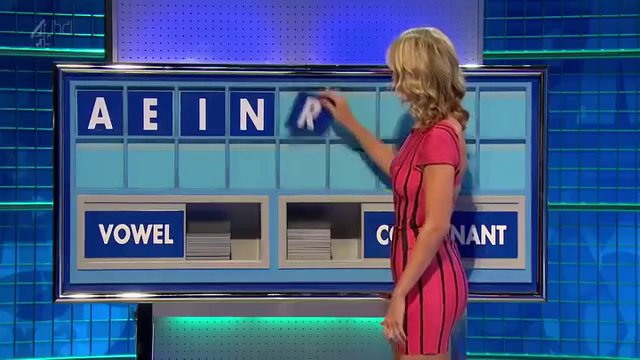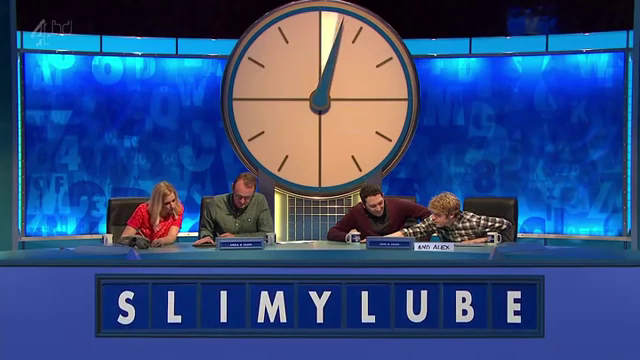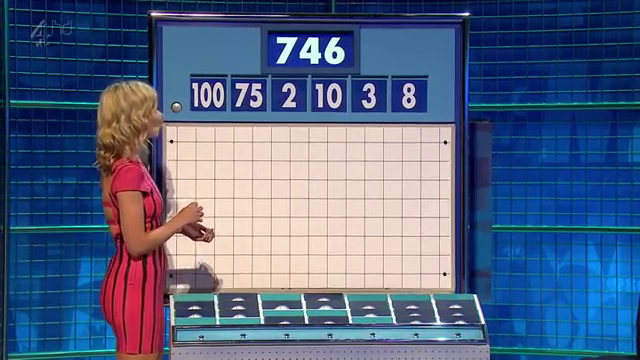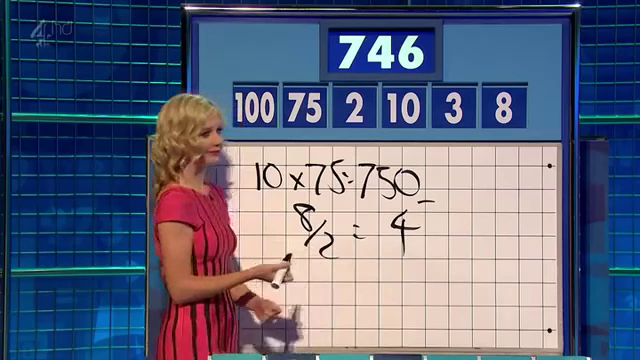Published: Mon 27 August 2018

I love watching 8 Out Of 10 Cats Does Countdown. It's a british panel show where a few comedians solve word puzzles and mathematics puzzles. It's based on the game show Countdown that's been running since 1982.

And I also like the host Jimmy Carr very much.

## The Puzzles

The show consists of three different puzzles:

In the Letters round a team (or single contestant) picks 9 consonants and vowels from their respective piles. After the team chooses from one of both piles, the letter is revealed and put up on the board. They then choose again until 9 letters are on the board. After all letters are on the board, the teams have 30 seconds to form a word out of as many of the letters as possible. The team with the longest word scores that many points.Letters Round

In the Conundrum a list of letters that already spell out a funny group of words is given by the host and the contestants try to find a 9 letter anagram. The first team to buzzer in the correct answer gains 10 points. This is the final round of the game.Conundrum

In the Numbers round a team (or single contestant) picks 6 numbers from two piles. A random number is picked and the contestants have to create a mathematical formula out of the picked numbers that equal the target number. The specific rules follow.Numbers Round

## Numbers Round

According to Wikipedia there are 6 columns of 4 numbers (4 rows). One column contains the numbers 25, 50, 75, and 100 and the other 5 columns contain the numbers 1 to 10 twice each. That's not exactly the same as on the show, as there appear to be 7 columns of 4 numbers but not every stack has all 4 number cards.

Contestants choose a total of 6 numbers from the "small" and "large" columns. The usual pick would be 2 to 3 "large" numbers and the rest small ones.

Then a random number between 100 und 999 is generated. The contestants try to create a mathematical formula that has the random number as the result. In the formulare only the following operations are allowed:

• Multiplication
• Subtraction (that does not result in a negative number)
• Division (that results in an integer)

The contestants do not have to use all the numbers to get the target number.Finished Numbers Round

Since I am really not good at this, I wrote a solver for the numbers game in Haskell.

## Solver

How do we go about this problem? First of all, we have to decide on a data structure. Let's start with a traditional term structure where a Term is a binary operation and two terms, or an integer value. An Operation is the function mapping two integers to a third, and a string to pretty-print the operation. The mapping is not total and might fail.

data Term = Op Operation Term Term | Val Integer
data Operation = Operation {fun :: (Integer -> Integer -> Maybe Integer), name :: String}

instance Show Term where
show (Val x) = show x
show (Op op l r) = "(" ++ (show l) ++ (name op) ++ show r ++ ")"


Now we have to define our operations and what they should do. Since we're using Maybe, and it's a monad, we can use monadic notation where it suits us:

operations = [  Operation (\x y -> return (x + y)) "+"
,  Operation (\x y -> return (x * y)) "*"
,  Operation (\x y -> guard (x > y) >> return (x - y)) "-"
,  Operation (\x y -> guard (x mod y == 0) >> return (x div y)) "/"
]
eval :: Term -> Maybe Integer
eval (Val v) = Just v
eval (Op o l r) = do l' <- eval l
r' <- eval r
(fun o) l' r'


As we can see, the preconditions to the subtraction and division are encoded using the guard function. If the function returns true, the whole operation returns Nothing, otherwise it returns Just the value.

Since Haskell uses lazy evaluation, we can define a list of all possible terms given a list of integers and filter for the ones that evaluate to the correct number.

As we create a Term we have to be careful to not use any of the numbers again. So we will split our numbers in two sets. One that can be used in the left Term of an Operation and the rest can be used in the right Term. We will create all terms using our definition. A Term is either the Value of one of the numbers for that subterm, or an operation with some numbers reserved for the left term and some numbers reserved for the right term.

terms :: [Integer] -> [Term]
terms nums =  do  n <- nums -- choose a number
[Val n] -- one solution
++
do  op <- operations -- choose an operation
(l, r) <- subset_split nums -- choose a split
guard $l /= [] guard$ r /= []
ls <- (terms l) -- choose a term for the left side
guard (eval ls /= Nothing)
rs <- (terms r) -- choose a term for the right side
guard (eval rs /= Nothing)
[Op op ls rs] -- one solution


For performance reasons we already evaluate the generated subterms in order to check whether the operations are valid. There are a lot of terms that can not be evaluated and we want to throw them out as early as possible (or not even generate them).

We haven't defined subset_split yet. The idea is, to just split the list of numbers in two groups in every possible way:

subsets :: [Integer] -> [[Integer]]
subsets []  = [[]]
subsets (x:xs) = subsets xs ++ map (x:) (subsets xs)

subset_split nums = do l <- subsets nums
return (l, nums \\ l)


Now all that remains is to generate all terms given a set of numbers, evaluate them, filter out the ones that don't have the correct value, and return the first one:

solve :: [Integer] -> Integer -> Maybe Term
solve nums target = let possible_solutions = map (\x -> (x, eval x)) (terms nums)
solutions = filter (\x -> (snd x) == (Just target)) possible_solutions
in listToMaybe $map fst solutions  And in our main we only read the list of numbers and the target as arguments and print the solution: main = do args <- getArgs let nums = (read (args !! 0))::[Integer] target = (read (args !! 1))::Integer print$ solve nums target


And there we go:

\$ ./countdown "[100, 75, 2, 10, 3, 8]" 746
Just (8+(3+((75*(100-2))/10)))


Our solution is $$8+3+{{75*(100-2)} \over 10}$$ while the solution from the show was $$10*75 - {8 \over 2}$$.

As you can see, we do not always get the easiest solution but we usually have a solution quite fast. This one took about a tenth of a second. The 30 seconds given to the contestant are usually quite enough. To make sure we had the "nicest" solution, we probably would need to generate all terms and sort them by size. The smallest one should be the nicest one.

Comments and Discussion is provided by Disqus. They are tracking site and user interaction. Please refer to their privacy policy for information about data usage and retention. If you still want to look at comments or comment yourself, enable disqus by clicking here.